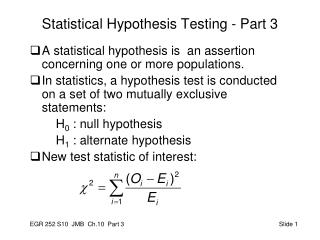DownloadDownload PresentationStatistical Hypothesis Testing - Part 3

# Statistical Hypothesis Testing - Part 3

Download Presentation## Statistical Hypothesis Testing - Part 3

- - - - - - - - - - - - - - - - - - - - - - - - - - - E N D - - - - - - - - - - - - - - - - - - - - - - - - - - -
##### Presentation Transcript

1. Statistical Hypothesis Testing - Part 3 • A statistical hypothesis is an assertion concerning one or more populations. • In statistics, a hypothesis test is conducted on a set of two mutually exclusive statements: H0 : null hypothesis H1 : alternate hypothesis • New test statistic of interest:

2. Goodness-of-Fit Tests • Procedures for confirming or refuting hypotheses about the distributions of random variables. • Hypotheses: H0: The population follows a particular distribution. H1: The population does not follow the distribution. Example: H0: The data come from a normal distribution. H1: The data do not come from a normal distribution.

3. Goodness of Fit Tests (cont.) • Test statistic is χ2 • Draw the picture • Determine the critical value for goodness of fit test χ2 with parameters α, ν = k – 1 • Calculate χ2 from the sample • Compare χ2calcto χ2crit • Make a decision about H0 • State your conclusion. • Discussion: Look at Table 10.4 in text.

4. Tests of Independence (without computer) • Example: Worker type and Choice of pension plan • Hypotheses H0: Pension Plan Choice and Worker Type are independent H1: Pension Plan Choice and Worker Type are not independent 1. Develop a Contingency Table of Observed Values

5. Worker vs. Pension Plan Example 2. Calculate expected probabilities. Multiply by total observations to determine expected values for each cell. P(#1 ∩ S) = P(#1)*P(S) = (200/500)*(340/500)=0.272 0.272*500 = 136 P(#1 ∩ H) = P(#1)*P(H) = (200/500)*(160/500)=0.128 0.128*500 = 64

6. Hypotheses Recall the general format of the hypotheses H0: the categories (worker & plan) are independent H1: the categories are not independent 3. Calculate the sample-based statistic (160-136)^2/136 + (140-136)^2/136 + (40-68)^2/68 + (40-64)^2/64 + (60-64)^2/64 + (60-32)^2/32 = 49.63

7. The Chi-Squared Test of Independence 4. Compare to the critical statistic for a test of independence, χ2α, r where r = (a – 1)(b – 1) a = # of columns b = # of rows For our example, let’s use α = 0.01 _ χ20.01,2 = 9.210 (from Table A.5, pp 756) Comparison: χ2 calc > χ2 crit Decision: Reject the null hypothesis Conclusion: Worker and plan are not independent. ****Note that we are stating that there is an association between the two categories. We are not claiming a cause and effect relationship.

8. Chi-Square Test Using Minitab Minitab Input: Minitab Output: Chi-Square Test: plan1, plan2, plan3 Expected counts are printed below observed counts Chi-Square contributions are printed below expected counts plan1 plan2 plan3 Total 1 160 140 40 340 136.00 136.00 68.00 4.235 0.118 11.529 2 40 60 60 160 64.00 64.00 32.00 9.000 0.250 24.500 Total 200 200 100 500 Chi-Sq = 49.632, DF = 2, P-Value = 0.000